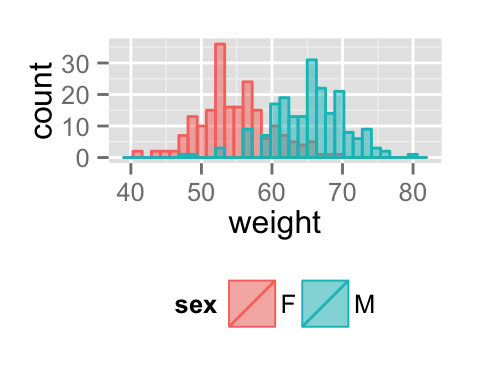# ggplot2 histogram plot : Quick start guide - R software and data visualization

This R tutorial describes how to create a histogram plot using R software and ggplot2 package.

The function geom_histogram() is used. You can also add a line for the mean using the function geom_vline.# Prepare the data

The data below will be used :

``````set.seed(1234)
df <- data.frame(
sex=factor(rep(c("F", "M"), each=200)),
weight=round(c(rnorm(200, mean=55, sd=5), rnorm(200, mean=65, sd=5)))
)
``````##   sex weight
## 1   F     49
## 2   F     56
## 3   F     60
## 4   F     43
## 5   F     57
## 6   F     58``````

# Basic histogram plots

``````library(ggplot2)
# Basic histogram
ggplot(df, aes(x=weight)) + geom_histogram()
# Change the width of bins
ggplot(df, aes(x=weight)) +
geom_histogram(binwidth=1)
# Change colors
p<-ggplot(df, aes(x=weight)) +
geom_histogram(color="black", fill="white")
p``````# Add mean line and density plot on the histogram

• The histogram is plotted with density instead of count on y-axis
• Overlay with transparent density plot. The value of alpha controls the level of transparency
``````# Add mean line
p+ geom_vline(aes(xintercept=mean(weight)),
color="blue", linetype="dashed", size=1)
# Histogram with density plot
ggplot(df, aes(x=weight)) +
geom_histogram(aes(y=..density..), colour="black", fill="white")+
geom_density(alpha=.2, fill="#FF6666") ``````Read more on ggplot2 line types : ggplot2 line types

# Change histogram plot line types and colors

``````# Change line color and fill color
ggplot(df, aes(x=weight))+
geom_histogram(color="darkblue", fill="lightblue")
# Change line type
ggplot(df, aes(x=weight))+
geom_histogram(color="black", fill="lightblue",
linetype="dashed")``````# Change histogram plot colors by groups

## Calculate the mean of each group :

The package plyr is used to calculate the average weight of each group :

``````library(plyr)
mu <- ddply(df, "sex", summarise, grp.mean=mean(weight))
``````##   sex grp.mean
## 1   F    54.70
## 2   M    65.36``````

## Change line colors

Histogram plot line colors can be automatically controlled by the levels of the variable sex.

Note that, you can change the position adjustment to use for overlapping points on the layer. Possible values for the argument position are “identity”, “stack”, “dodge”. Default value is “stack”.

``````# Change histogram plot line colors by groups
ggplot(df, aes(x=weight, color=sex)) +
geom_histogram(fill="white")
# Overlaid histograms
ggplot(df, aes(x=weight, color=sex)) +
geom_histogram(fill="white", alpha=0.5, position="identity")````````````# Interleaved histograms
ggplot(df, aes(x=weight, color=sex)) +
geom_histogram(fill="white", position="dodge")+
theme(legend.position="top")
p<-ggplot(df, aes(x=weight, color=sex)) +
geom_histogram(fill="white", position="dodge")+
geom_vline(data=mu, aes(xintercept=grp.mean, color=sex),
linetype="dashed")+
theme(legend.position="top")
p``````It is also possible to change manually histogram plot line colors using the functions :

• scale_color_manual() : to use custom colors
• scale_color_brewer() : to use color palettes from RColorBrewer package
• scale_color_grey() : to use grey color palettes
``````# Use custom color palettes
p+scale_color_manual(values=c("#999999", "#E69F00", "#56B4E9"))
# Use brewer color palettes
p+scale_color_brewer(palette="Dark2")
# Use grey scale
p + scale_color_grey() + theme_classic() +
theme(legend.position="top")``````Read more on ggplot2 colors here : ggplot2 colors

## Change fill colors

Histogram plot fill colors can be automatically controlled by the levels of sex :

``````# Change histogram plot fill colors by groups
ggplot(df, aes(x=weight, fill=sex, color=sex)) +
geom_histogram(position="identity")
# Use semi-transparent fill
p<-ggplot(df, aes(x=weight, fill=sex, color=sex)) +
geom_histogram(position="identity", alpha=0.5)
p
p+geom_vline(data=mu, aes(xintercept=grp.mean, color=sex),
linetype="dashed")``````It is also possible to change manually histogram plot fill colors using the functions :

• scale_fill_manual() : to use custom colors
• scale_fill_brewer() : to use color palettes from RColorBrewer package
• scale_fill_grey() : to use grey color palettes
``````# Use custom color palettes
p+scale_color_manual(values=c("#999999", "#E69F00", "#56B4E9"))+
scale_fill_manual(values=c("#999999", "#E69F00", "#56B4E9"))
# use brewer color palettes
p+scale_color_brewer(palette="Dark2")+
scale_fill_brewer(palette="Dark2")
# Use grey scale
p + scale_color_grey()+scale_fill_grey() +
theme_classic()``````Read more on ggplot2 colors here : ggplot2 colors

# Change the legend position

``````p + theme(legend.position="top")
p + theme(legend.position="bottom")
# Remove legend
p + theme(legend.position="none")``````The allowed values for the arguments legend.position are : “left”,“top”, “right”, “bottom”.

Read more on ggplot legends : ggplot2 legends

# Use facets

Split the plot into multiple panels :

``````p<-ggplot(df, aes(x=weight))+
geom_histogram(color="black", fill="white")+
facet_grid(sex ~ .)
p
p+geom_vline(data=mu, aes(xintercept=grp.mean, color="red"),
linetype="dashed")``````Read more on facets : ggplot2 facets

# Customized histogram plots

``````# Basic histogram
ggplot(df, aes(x=weight, fill=sex)) +
geom_histogram(fill="white", color="black")+
geom_vline(aes(xintercept=mean(weight)), color="blue",
linetype="dashed")+
labs(title="Weight histogram plot",x="Weight(kg)", y = "Count")+
theme_classic()
# Change line colors by groups
ggplot(df, aes(x=weight, color=sex, fill=sex)) +
geom_histogram(position="identity", alpha=0.5)+
geom_vline(data=mu, aes(xintercept=grp.mean, color=sex),
linetype="dashed")+
scale_color_manual(values=c("#999999", "#E69F00", "#56B4E9"))+
scale_fill_manual(values=c("#999999", "#E69F00", "#56B4E9"))+
labs(title="Weight histogram plot",x="Weight(kg)", y = "Count")+
theme_classic()``````Combine histogram and density plots :

``````# Change line colors by groups
ggplot(df, aes(x=weight, color=sex, fill=sex)) +
geom_histogram(aes(y=..density..), position="identity", alpha=0.5)+
geom_density(alpha=0.6)+
geom_vline(data=mu, aes(xintercept=grp.mean, color=sex),
linetype="dashed")+
scale_color_manual(values=c("#999999", "#E69F00", "#56B4E9"))+
scale_fill_manual(values=c("#999999", "#E69F00", "#56B4E9"))+
labs(title="Weight histogram plot",x="Weight(kg)", y = "Density")+
theme_classic()``````Change line colors manually :

``````p<-ggplot(df, aes(x=weight, color=sex)) +
geom_histogram(fill="white", position="dodge")+
geom_vline(data=mu, aes(xintercept=grp.mean, color=sex),
linetype="dashed")
# Continuous colors
p + scale_color_brewer(palette="Paired") +
theme_classic()+theme(legend.position="top")
# Discrete colors
p + scale_color_brewer(palette="Dark2") +
theme_minimal()+theme_classic()+theme(legend.position="top")
p + scale_color_brewer(palette="Accent") +
theme_minimal()+theme(legend.position="top")``````Read more on ggplot2 colors here : ggplot2 colors

# Infos

This analysis has been performed using R software (ver. 3.1.2) and ggplot2 (ver. 1.0.0)